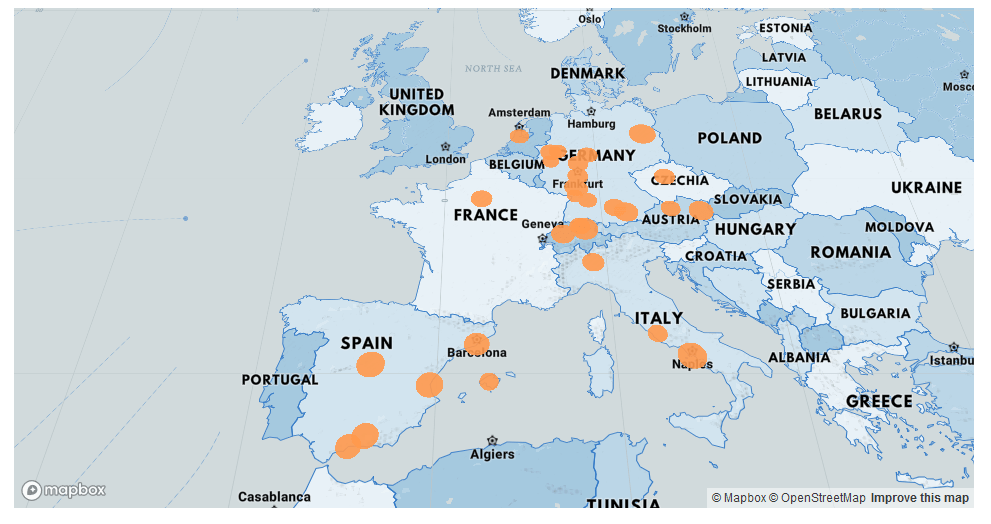# Map-based scatter plots with deckgl in RIn this blogpost I provide a coding example in R for how to create a map-based scatterplot using the deckgl package. This can come in handy when visualising data with some spatial aspect. For example you might want to visualise the geo-spatial distribution of certain property clusters.

Before I can apply the deckgl package’s functionality I need a geocoded dataset, i.e. a dataset which contains information about longitude and latitude coordinates of the property of interest. For this I will use a geocoding function applying the Open Street Map API. I found the function on datascienceplus.com.

``````# osm geocoder
{
return(data.frame())

tryCatch(
d <- jsonlite::fromJSON(
), error = function(c) return(data.frame())
)

if(length(d) == 0)
return(data.frame())

return(data.frame(lon = as.numeric(d\$lon), lat = as.numeric(d\$lat)))
}``````

Next, I need to initialize the data I want to plot. I stored a list of cities in a separate csv-file. I thus read in this file and convert it into a dataframe. I then use the geocoding function to geocode all the cities in my data frame. In addition I add normally distributed values to the “entries” and “exits” column; required for determining e.g. circle design in the scatterplot.

``````# ensuring that required packages are loaded
library(deckgl)``````
``````## deckgl 0.1.8 wrapping deckgljs 6.2.4
##   Documentation: https://crazycapivara.github.io/deckgl/
##   Issues, notes and bleeding edge: https://github.com/crazycapivara/deckgl``````
``````library(magrittr)
library(jsonlite)
library(dplyr)``````
``````##
## Attaching package: 'dplyr'``````
``````## The following objects are masked from 'package:stats':
##
##     filter, lag``````
``````## The following objects are masked from 'package:base':
##
##     intersect, setdiff, setequal, union``````
``````# setting up the data frames
scatter_data_df_1 <- data.frame(matrix(nrow=30,ncol=6))

colnames(scatter_data_df_1) <- column_names

# geocode cities into longitude and latitude
for(i in 1:nrow(city_list_1_df)){
dum_coord <- osm_geocoder(toString(city_list_1_df\$V1[i]))
scatter_data_df_1\$name[i] <- paste0("city liste 1 : ",i)
scatter_data_df_1\$code[i] <- c("CL1")
scatter_data_df_1\$entries[i] <- as.integer(rnorm(1,mean=3000,sd=1000))
scatter_data_df_1\$exits[i] <- as.integer(rnorm(1,mean=3000,1000))
scatter_data_df_1\$coordinates[i] <- list(c(as.numeric(dum_coord),as.numeric(dum_coord)))
}

``````##               name code            address entries exits
## 1 city liste 1 : 1  CL1     Berlin Germany    5008  3112
## 2 city liste 1 : 2  CL1  Karlsruhe Germany    2002  2223
## 3 city liste 1 : 3  CL1  Stuttgart Germany    3453  3498
## 4 city liste 1 : 4  CL1   Mannheim Germany    2478  3041
## 5 city liste 1 : 5  CL1 Heidelberg Germany    3811  1003
## 6 city liste 1 : 6  CL1  Frankfurt Germany    1875  3135
##           coordinates
## 1  13.38886, 52.51704
## 2   8.40342, 49.00687
## 3 9.180013, 48.778449
## 4 8.467236, 49.489591
## 5 8.694724, 49.409358
## 6 8.682092, 50.110644``````

I can now create the scatterplot, using the deckgl function from the deckgl R-package.

``````# define properties of the plot
properties_1 <- list(
getPosition = get_property("coordinates"),
getColor = c(255, 153, 77)
)

# plot scatterplot
deckgl(zoom = 10.5, pitch = 35, longitude = 8.40342, latitude = 40.00687) %>%
add_scatterplot_layer(data = scatter_data_df_1, properties = properties_1) %>%